# RD Sharma Solutions Class 9 Maths Chapter 21 Surface Area And Volume Of Sphere Exercise 21.2

Class 9 Chapter 21 – Surface Area and Volume of A Sphere Exercise 21.2 solutions are provided here. These questions and answers are designed by the experts of Maths. This exercise is based on volume of a sphere, hemisphere and spherical shell. RD Sharma class 9 solutions will help students in constructive preparation and learning. Students can practice all the questions and match their solution given below. You can also download exercise 21.2 pdf for future reference by following the given link.

## Download PDF of RD Sharma Solutions for Class 9 Maths Chapter 21 Surface Area and Volume of A Sphere Exercise 21.2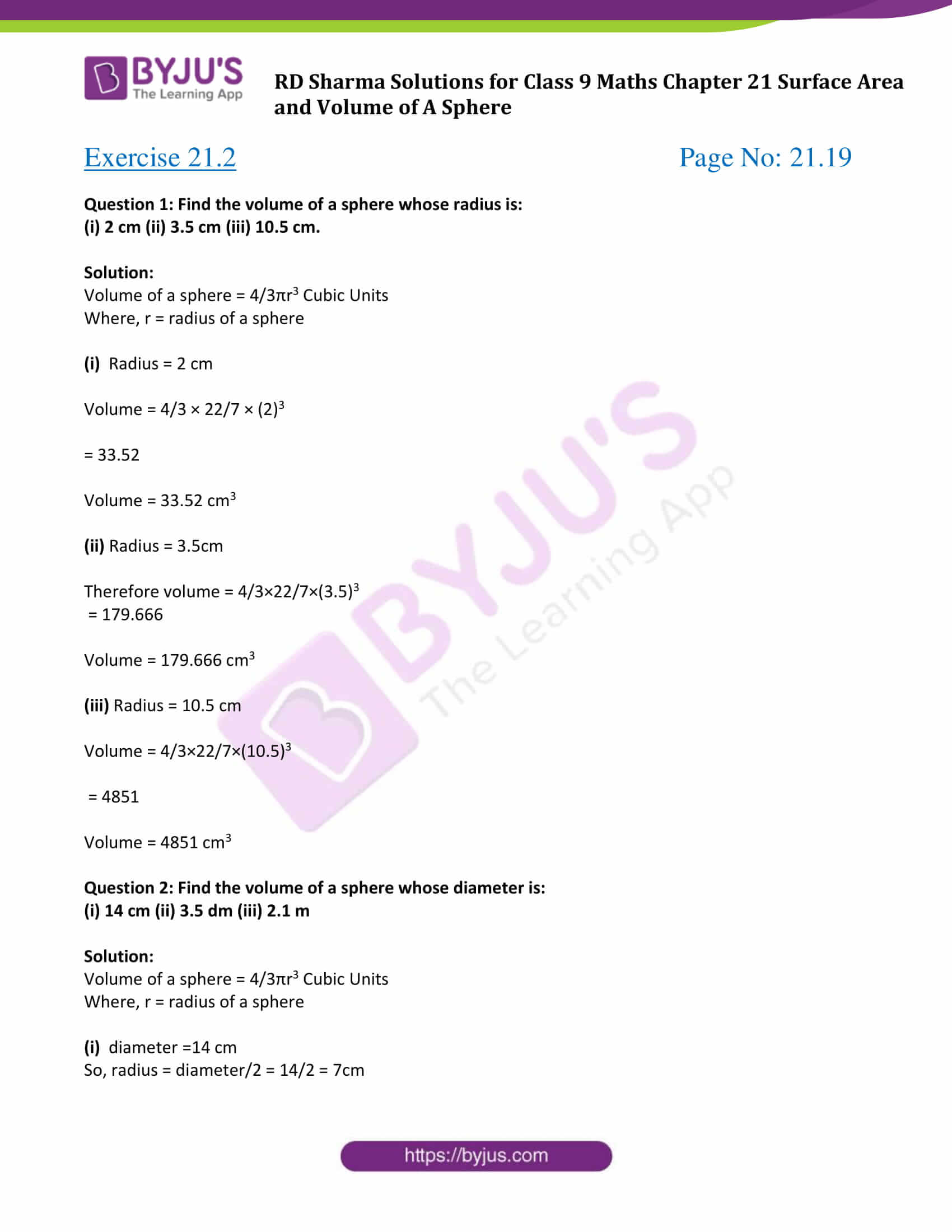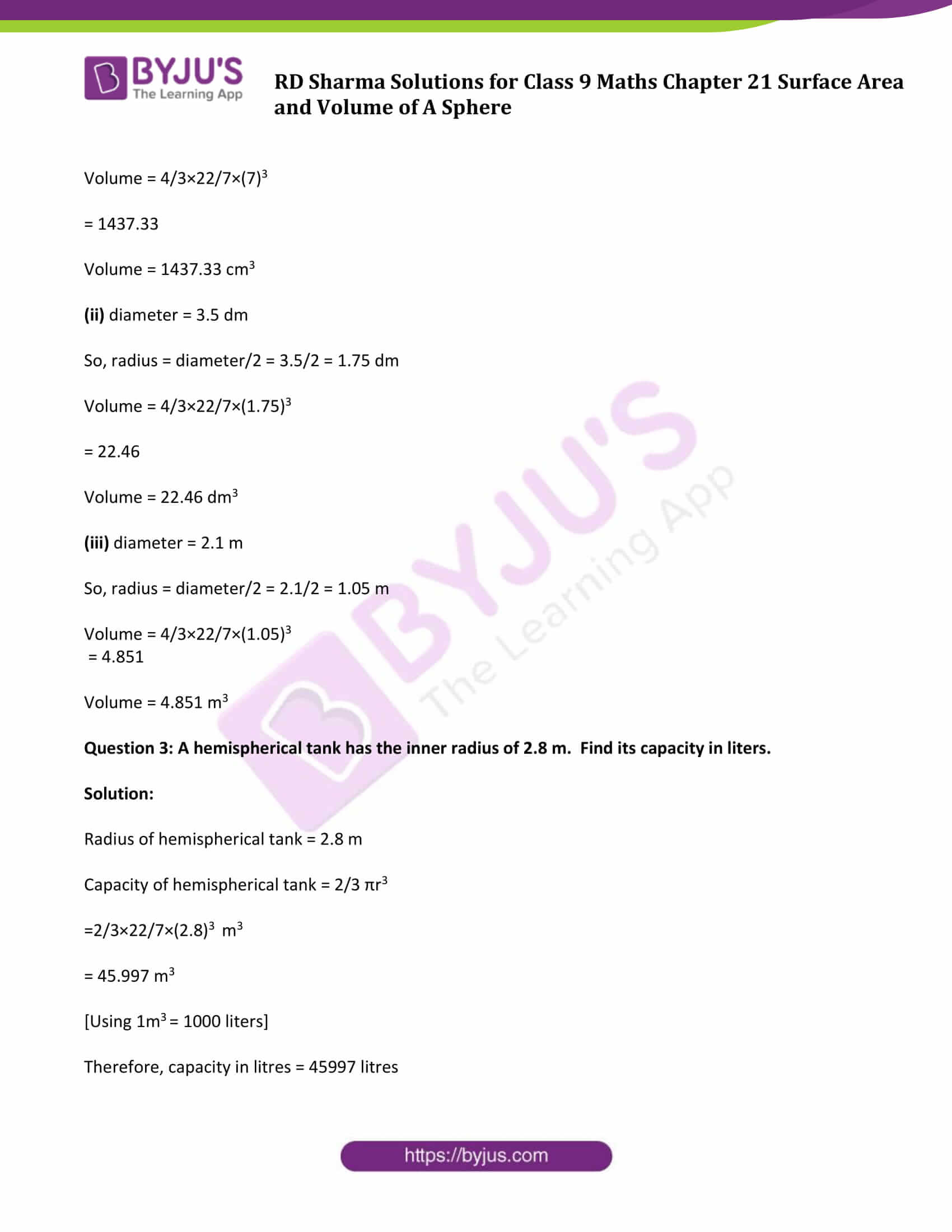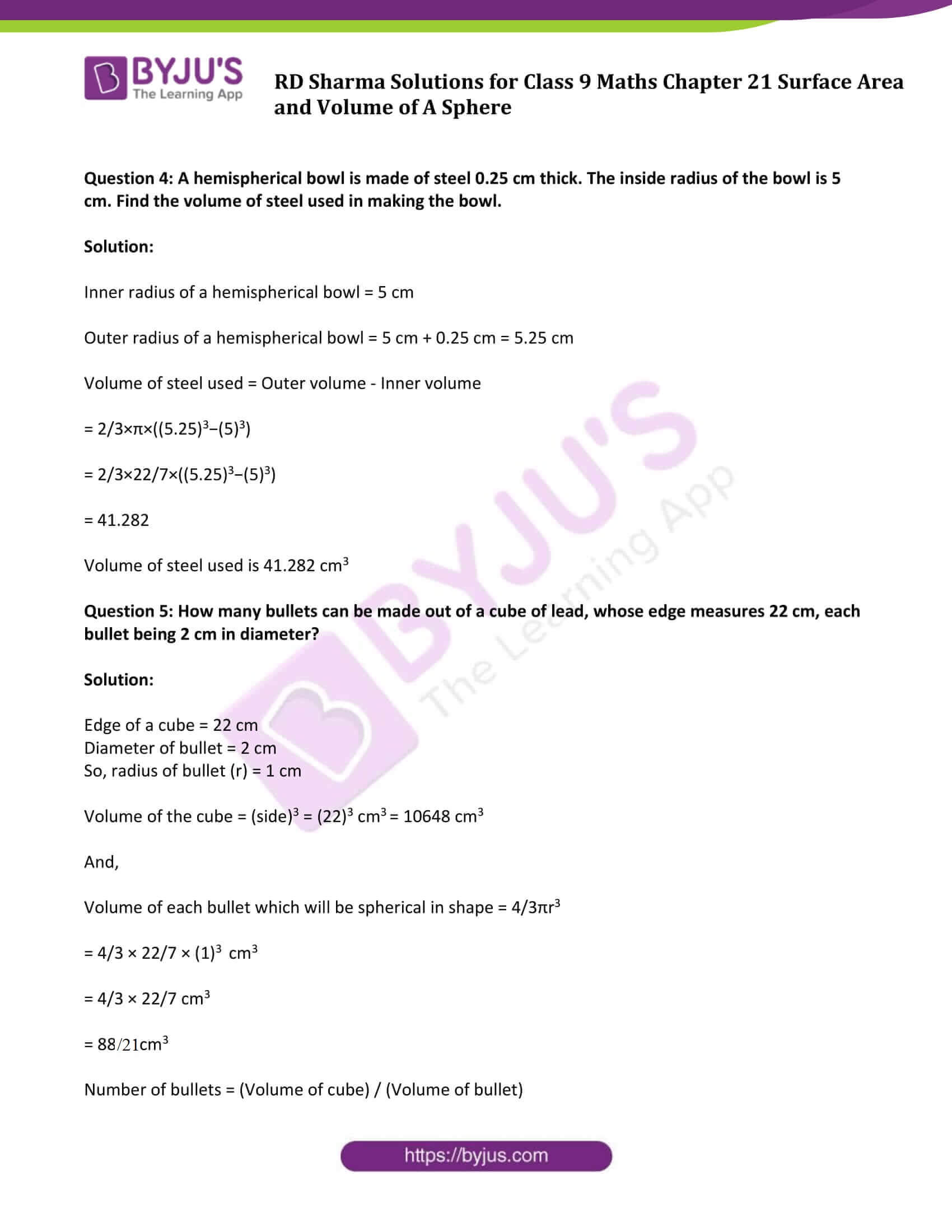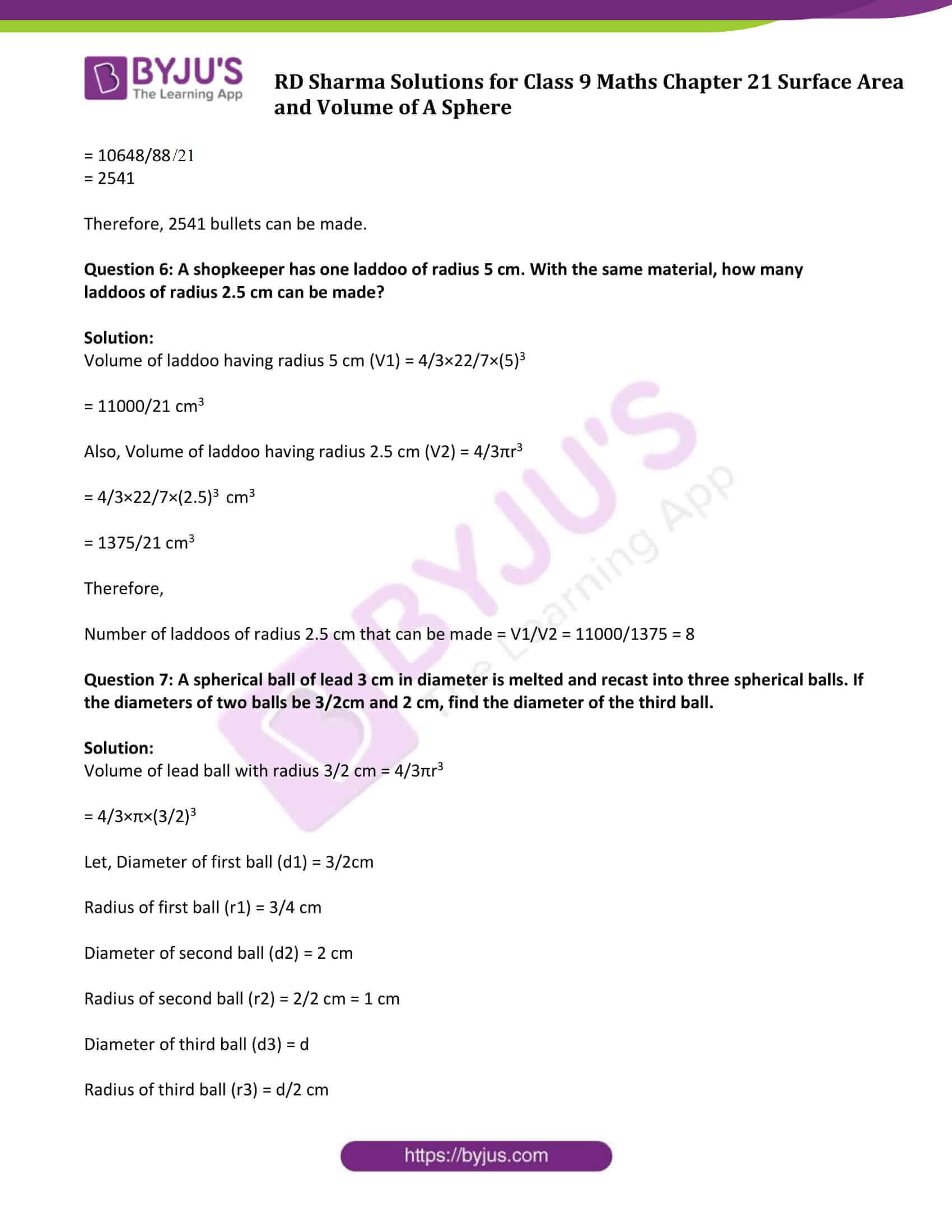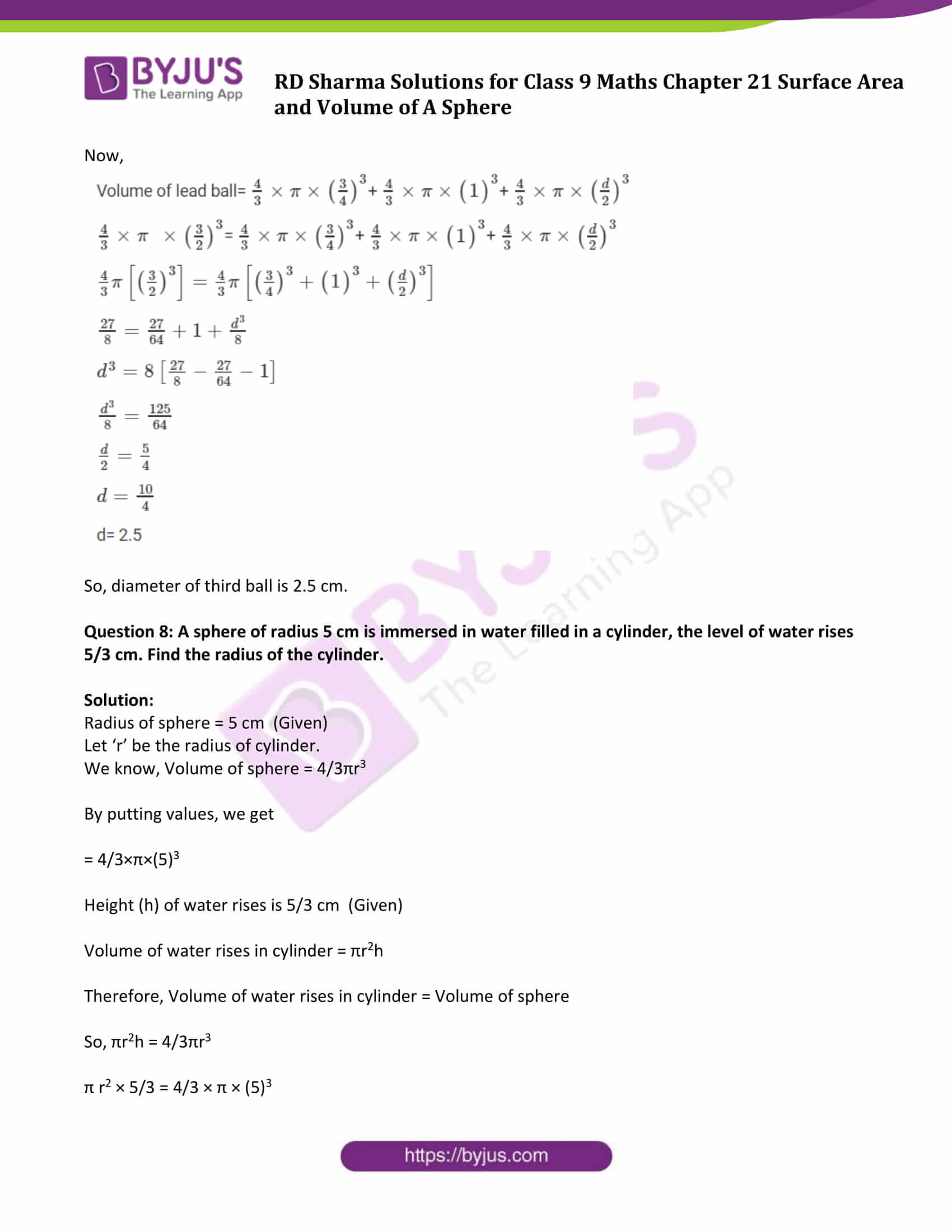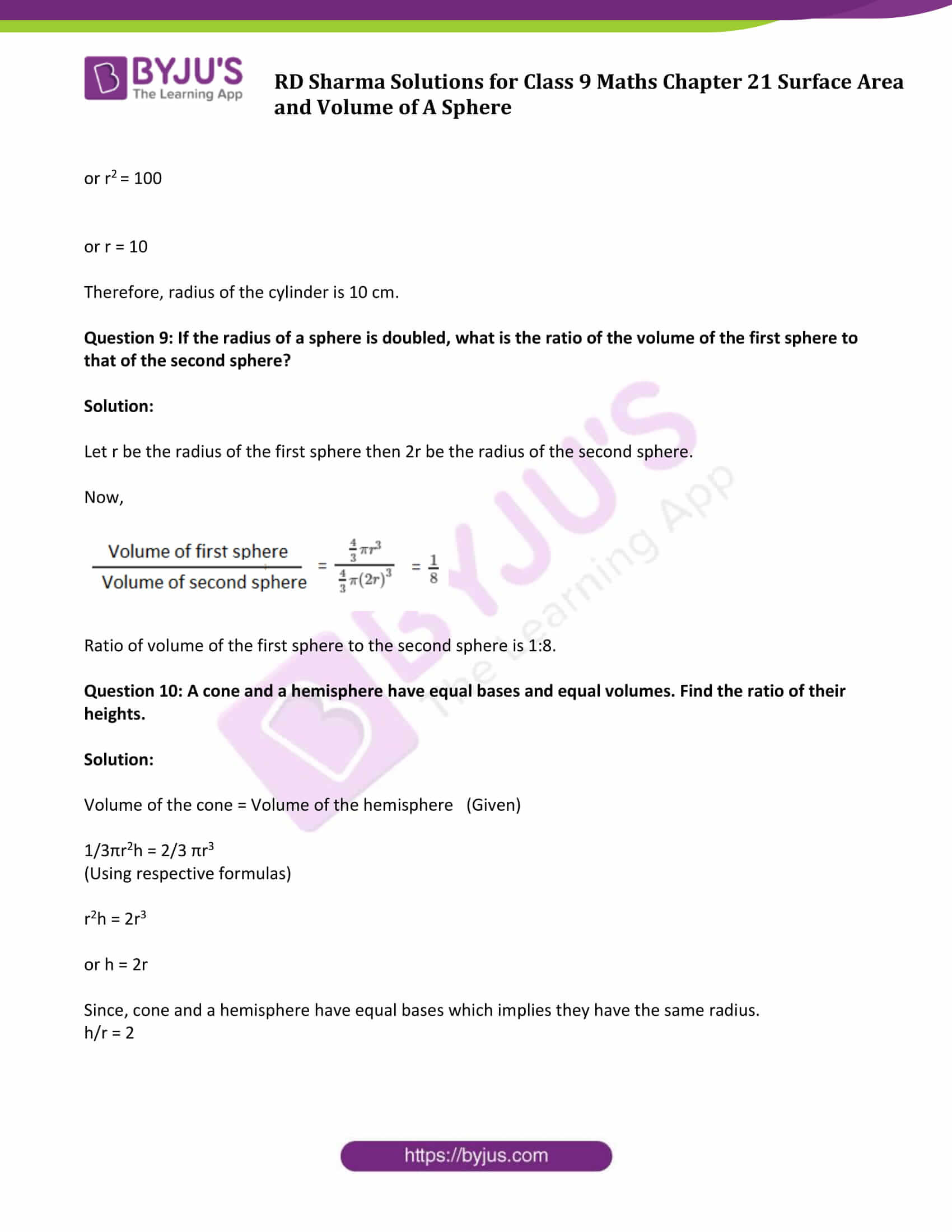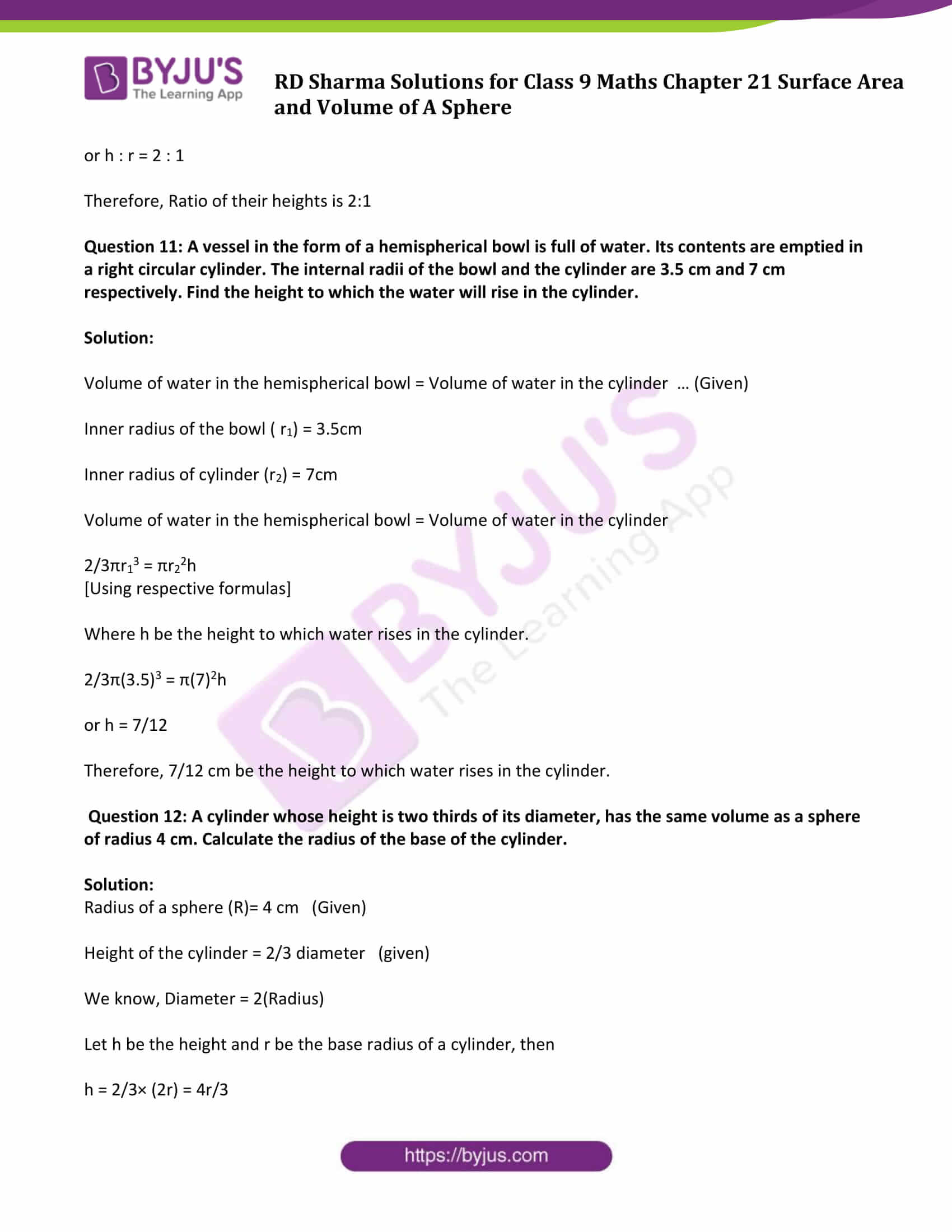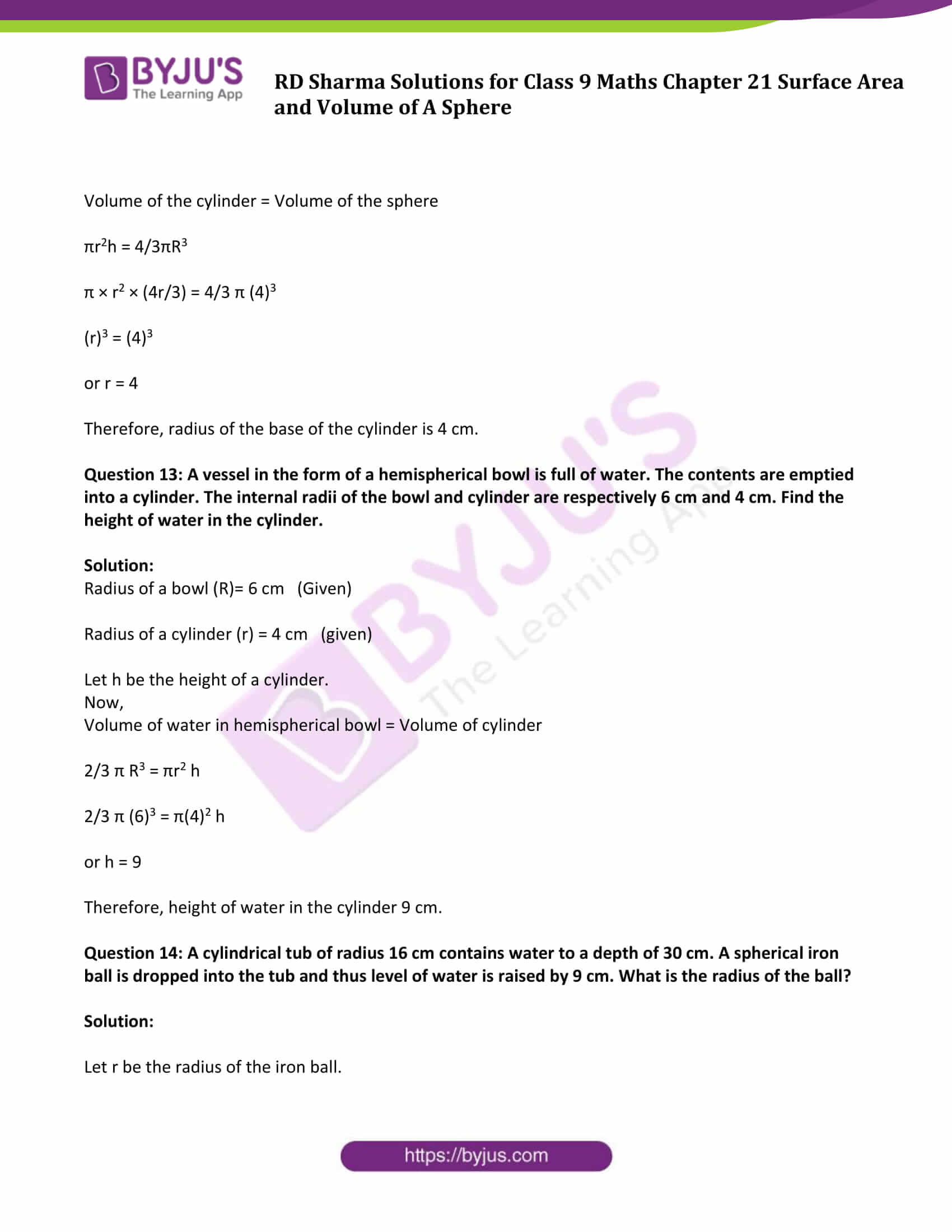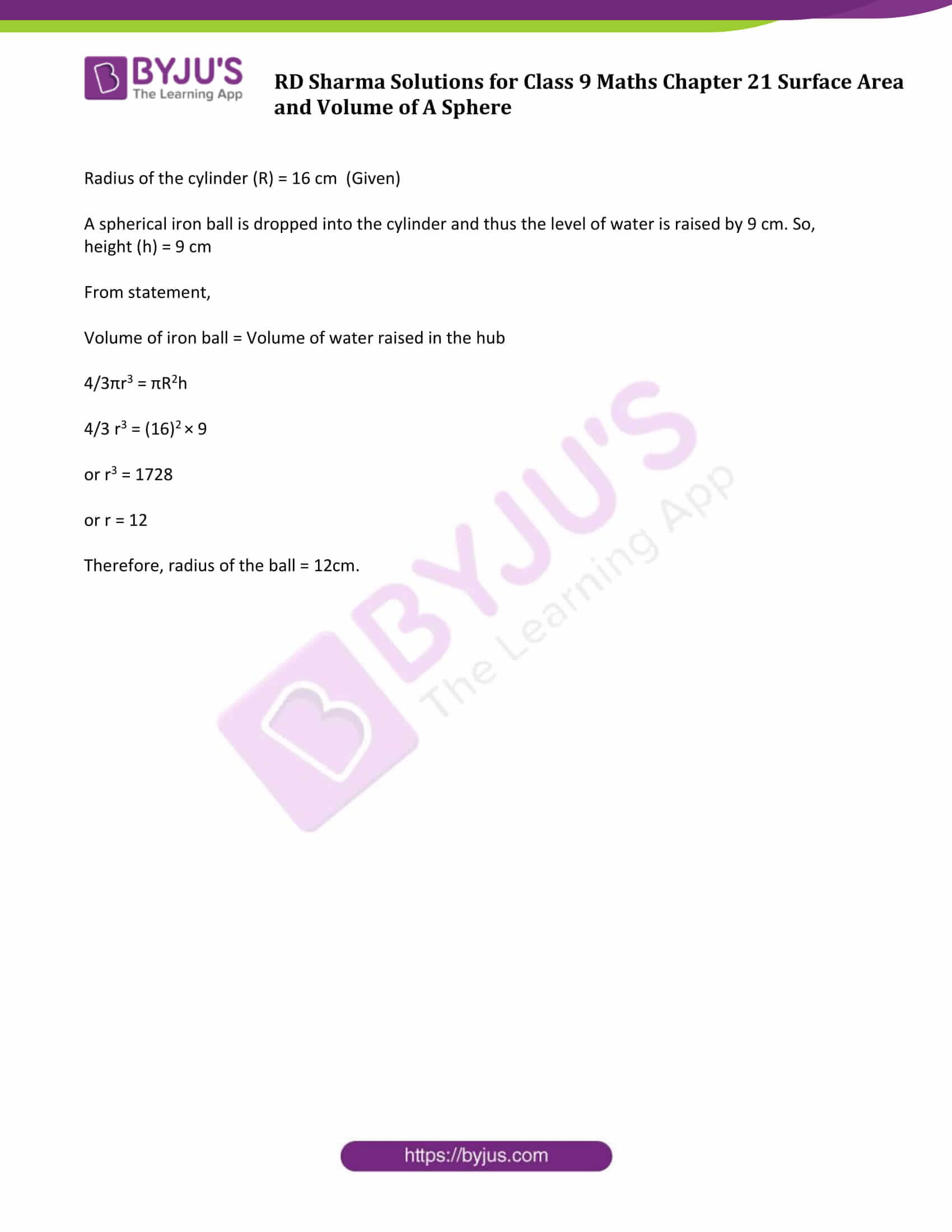### Access Answers to Maths RD Sharma Solutions for Class 9 Chapter 21 Surface Area and Volume of A Sphere Exercise 21.2 Page number 21.19

Question 1: Find the volume of a sphere whose radius is:

(i) 2 cm (ii) 3.5 cm (iii) 10.5 cm.

Solution:

Volume of a sphere = 4/3πr3 Cubic Units

Where, r = radius of a sphere

Volume = 4/3 × 22/7 × (2)3

= 33.52

Volume = 33.52 cm3

Therefore volume = 4/3×22/7×(3.5)3

= 179.666

Volume = 179.666 cm3

Volume = 4/3×22/7×(10.5)3

= 4851

Volume = 4851 cm3

Question 2: Find the volume of a sphere whose diameter is:

(i) 14 cm (ii) 3.5 dm (iii) 2.1 m

Solution:

Volume of a sphere = 4/3πr3 Cubic Units

Where, r = radius of a sphere

(i) diameter =14 cm

So, radius = diameter/2 = 14/2 = 7cm

Volume = 4/3×22/7×(7)3

= 1437.33

Volume = 1437.33 cm3

(ii) diameter = 3.5 dm

So, radius = diameter/2 = 3.5/2 = 1.75 dm

Volume = 4/3×22/7×(1.75)3

= 22.46

Volume = 22.46 dm3

(iii) diameter = 2.1 m

So, radius = diameter/2 = 2.1/2 = 1.05 m

Volume = 4/3×22/7×(1.05)3

= 4.851

Volume = 4.851 m3

Question 3: A hemispherical tank has the inner radius of 2.8 m. Find its capacity in liters.

Solution:

Radius of hemispherical tank = 2.8 m

Capacity of hemispherical tank = 2/3 πr3

=2/3×22/7×(2.8)3 m3

= 45.997 m3

[Using 1m3 = 1000 liters]

Therefore, capacity in litres = 45997 litres

Question 4: A hemispherical bowl is made of steel 0.25 cm thick. The inside radius of the bowl is 5 cm. Find the volume of steel used in making the bowl.

Solution:

Inner radius of a hemispherical bowl = 5 cm

Outer radius of a hemispherical bowl = 5 cm + 0.25 cm = 5.25 cm

Volume of steel used = Outer volume – Inner volume

= 2/3×π×((5.25)3−(5)3)

= 2/3×22/7×((5.25)3−(5)3)

= 41.282

Volume of steel used is 41.282 cm3

Question 5: How many bullets can be made out of a cube of lead, whose edge measures 22 cm, each bullet being 2 cm in diameter?

Solution:

Edge of a cube = 22 cm

Diameter of bullet = 2 cm

So, radius of bullet (r) = 1 cm

Volume of the cube = (side)3 = (22)3 cm3 = 10648 cm3

And,

Volume of each bullet which will be spherical in shape = 4/3πr3

= 4/3 × 22/7 × (1)3 cm3

= 4/3 × 22/7 cm3

= 88/21 cm3

Number of bullets = (Volume of cube) / (Volume of bullet)

= 10648/88/21

= 2541

Therefore, 2541 bullets can be made.

Solution:

= 11000/21 cm3

= 4/3×22/7×(2.5)3 cm3

= 1375/21 cm3

Therefore,

Number of laddoos of radius 2.5 cm that can be made = V1/V2 = 11000/1375 = 8

Question 7: A spherical ball of lead 3 cm in diameter is melted and recast into three spherical balls. If the diameters of two balls be 3/2cm and 2 cm, find the diameter of the third ball.

Solution:

= 4/3×π×(3/2)3

Let, Diameter of first ball (d1) = 3/2cm

Radius of first ball (r1) = 3/4 cm

Diameter of second ball (d2) = 2 cm

Radius of second ball (r2) = 2/2 cm = 1 cm

Diameter of third ball (d3) = d

Radius of third ball (r3) = d/2 cm

Now,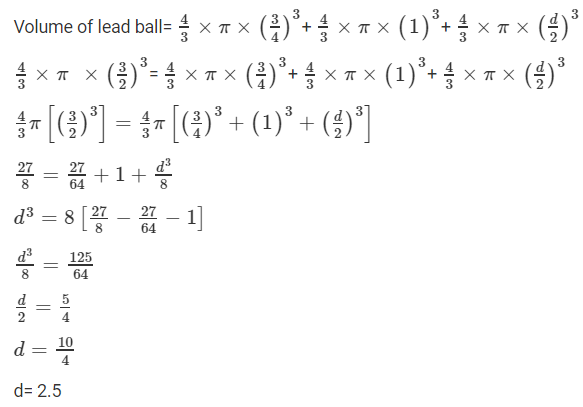So, diameter of third ball is 2.5 cm.

Question 8: A sphere of radius 5 cm is immersed in water filled in a cylinder, the level of water rises 5/3 cm. Find the radius of the cylinder.

Solution:

Radius of sphere = 5 cm (Given)

Let ‘r’ be the radius of cylinder.

We know, Volume of sphere = 4/3πr3

By putting values, we get

= 4/3×π×(5)3

Height (h) of water rises is 5/3 cm (Given)

Volume of water rises in cylinder = πr2h

Therefore, Volume of water rises in cylinder = Volume of sphere

So, πr2h = 4/3πr3

π r2 × 5/3 = 4/3 × π × (5)3

or r2 = 100

or r = 10

Therefore, radius of the cylinder is 10 cm.

Question 9: If the radius of a sphere is doubled, what is the ratio of the volume of the first sphere to that of the second sphere?

Solution:

Let r be the radius of the first sphere then 2r be the radius of the second sphere.

Now,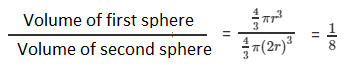Ratio of volume of the first sphere to the second sphere is 1:8.

Question 10: A cone and a hemisphere have equal bases and equal volumes. Find the ratio of their heights.

Solution:

Volume of the cone = Volume of the hemisphere (Given)

1/3πr2h = 2/3 πr3

(Using respective formulas)

r2h = 2r3

or h = 2r

Since, cone and a hemisphere have equal bases which implies they have the same radius.

h/r = 2

or h : r = 2 : 1

Therefore, Ratio of their heights is 2:1

Question 11: A vessel in the form of a hemispherical bowl is full of water. Its contents are emptied in a right circular cylinder. The internal radii of the bowl and the cylinder are 3.5 cm and 7 cm respectively. Find the height to which the water will rise in the cylinder.

Solution:

Volume of water in the hemispherical bowl = Volume of water in the cylinder … (Given)

Inner radius of the bowl ( r1) = 3.5cm

Inner radius of cylinder (r2) = 7cm

Volume of water in the hemispherical bowl = Volume of water in the cylinder

2/3πr13 = πr22h

[Using respective formulas]

Where h be the height to which water rises in the cylinder.

2/3π(3.5)3 = π(7)2h

or h = 7/12

Therefore, 7/12 cm be the height to which water rises in the cylinder.

Question 12: A cylinder whose height is two thirds of its diameter, has the same volume as a sphere of radius 4 cm. Calculate the radius of the base of the cylinder.

Solution:

Radius of a sphere (R)= 4 cm (Given)

Height of the cylinder = 2/3 diameter (given)

Let h be the height and r be the base radius of a cylinder, then

h = 2/3× (2r) = 4r/3

Volume of the cylinder = Volume of the sphere

πr2h = 4/3πR3

π × r2 × (4r/3) = 4/3 π (4)3

(r)3 = (4)3

or r = 4

Therefore, radius of the base of the cylinder is 4 cm.

Question 13: A vessel in the form of a hemispherical bowl is full of water. The contents are emptied into a cylinder. The internal radii of the bowl and cylinder are respectively 6 cm and 4 cm. Find the height of water in the cylinder.

Solution:

Radius of a bowl (R)= 6 cm (Given)

Radius of a cylinder (r) = 4 cm (given)

Let h be the height of a cylinder.

Now,

Volume of water in hemispherical bowl = Volume of cylinder

2/3 π R3 = πr2 h

2/3 π (6)3 = π(4)2 h

or h = 9

Therefore, height of water in the cylinder 9 cm.

Question 14: A cylindrical tub of radius 16 cm contains water to a depth of 30 cm. A spherical iron ball is dropped into the tub and thus level of water is raised by 9 cm. What is the radius of the ball?

Solution:

Let r be the radius of the iron ball.

Radius of the cylinder (R) = 16 cm (Given)

A spherical iron ball is dropped into the cylinder and thus the level of water is raised by 9 cm. So, height (h) = 9 cm

From statement,

Volume of iron ball = Volume of water raised in the hub

4/3πr3 = πR2h

4/3 r3 = (16)2 × 9

or r3 = 1728

or r = 12

Therefore, radius of the ball = 12cm.

## RD Sharma Solutions for Class 9 Maths Chapter 21 Surface Area and Volume of A Sphere Exercise 21.2

RD Sharma Solutions Class 9 Maths Chapter 21 Surface Area and Volume of A Sphere Exercise 21.2 is based on following topics:

• Volume of a sphere
• Volume of a hemisphere and
• Volume of a spherical shell